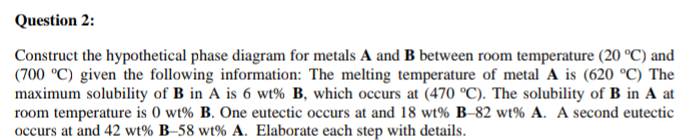# Question Solved1 AnswerQuestion 2: Construct the hypothetical phase diagram for metals A and B between room temperature (20 °C) and (700 °C) given the following information: The melting temperature of metal A is (620 °C) The maximum solubility of B in A is 6 wt% B, which occurs at (470 °C). The solubility of B in A at room temperature is O wt% B. One eutectic occurs at and 18 wt% B-82 wt% A. A second eutectic occurs at and 42 wt% B-58 wt% A. Elaborate each step with details.Transcribed Image Text: Question 2: Construct the hypothetical phase diagram for metals A and B between room temperature (20 °C) and (700 °C) given the following information: The melting temperature of metal A is (620 °C) The maximum solubility of B in A is 6 wt% B, which occurs at (470 °C). The solubility of B in A at room temperature is O wt% B. One eutectic occurs at and 18 wt% B-82 wt% A. A second eutectic occurs at and 42 wt% B-58 wt% A. Elaborate each step with details.
More
Transcribed Image Text: Question 2: Construct the hypothetical phase diagram for metals A and B between room temperature (20 °C) and (700 °C) given the following information: The melting temperature of metal A is (620 °C) The maximum solubility of B in A is 6 wt% B, which occurs at (470 °C). The solubility of B in A at room temperature is O wt% B. One eutectic occurs at and 18 wt% B-82 wt% A. A second eutectic occurs at and 42 wt% B-58 wt% A. Elaborate each step with details.